# 论文引入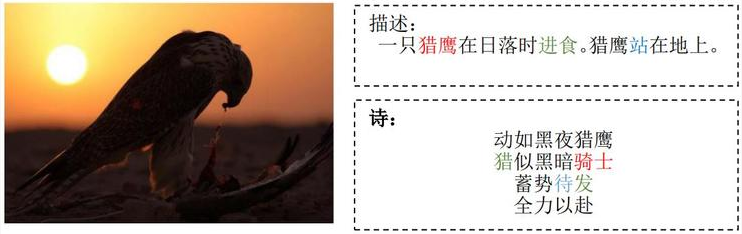img2poem主要关注的是一种开放形式的诗歌——自由诗。在不要求格律、韵律或其他传统的诗歌技术下，要有诗歌结构和诗歌语言，可以称之为诗意。 例如，诗歌的长度一般有限；与图像描述相比，诗歌一般偏好特定的词语；诗歌中的语句应与同一主题相关，保持一致。

img2poem的主要优势为：

• 提出以自动方式从图像生成诗歌（英文自由诗），这是首个尝试在整体框架中研究图像生成英文自由诗歌问题，它使机器在认知工作中能够具备接近人类的能力。
• 将深度耦合的视觉诗意嵌入模型与基于RNN的联合学习生成器结合，其中两个判别器通过多对抗训练，为跨模态相关性和诗意提供奖励。
• 收集了首个人类注解的图像-诗歌对数据集，以及最大的公共诗歌语料数据集。

# 模型框架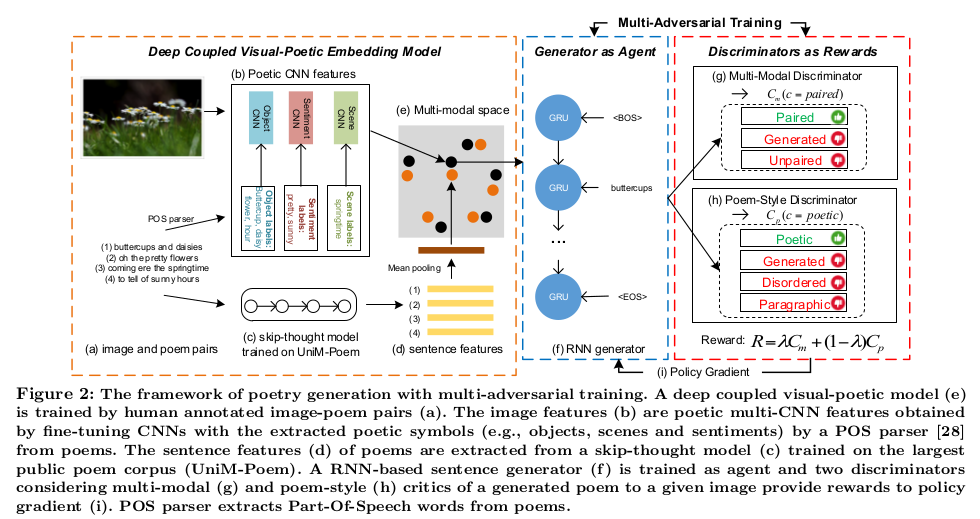$\begin{equation} loss = - \frac{1}{n} \sum_{n=1}^N (t_n log p_n + (1 - t_n)log(1 - p_n)) \end{equation}$

$v_1 = f_{Object}(I), v_2 = f_{Scene}(I), v_3 = f_{Sentiment}(I), v = (v_1, v_2, v_3).$

$\begin{equation} x = W_v \cdot v + b_v \end{equation}$

$\begin{equation} m = W_t \cdot t + b_t \end{equation}$

$L = \sum_x \sum_k max(0, \alpha - x \cdot m + x \cdot m_k) + \sum_m \sum_k max(0, \alpha - m \cdot x + m \cdot x_k)$

$\begin{equation} J(\theta) = \sum_{y_{1:T} \in \mathbb Y^*} p_{\theta} (y_{1:T}) R(y_{1:T}) = \mathbb E_{y_{1:T} \sim p_{\theta}} \sum_{t=1}^T r(y_{1:t}) \end{equation}$

$\begin{equation} \nabla_{\theta} J(\theta) = \mathbb E_{y_{1:T} \sim p_{\theta}} [\sum_{t=1}^T \nabla_{\theta} log p_{\theta} (y_{1:(t-1)})] \sum_{t=1}^T r(y_{1:t}) \end{equation}$

$\begin{equation} \nabla_{\theta} J(\theta) \approx \sum_{t=1}^T \nabla_{\theta} log p_{\theta} (y_{1:(t-1)}) \sum_{t=1}^T r(y_{1:t} - b_t) \end{equation}$

$\begin{equation} c = GRU_{\rho}(y) \\ f = tanh(W_x \cdot x + b_x) \odot tanh(W_c \cdot c + b_c) \\ C_m = softmax(W_m \cdot f + b_m) \end{equation}$

$\begin{equation} C_p = softmax(W_p \cdot GRU_{\eta}(y) + b_p) \end{equation}$

$\begin{equation} R(y \vert \cdot) = \lambda C_m (c = paired \vert x, y) + (1 - \lambda)C_p (c = poetic \vert y) \end{equation}$

# 实验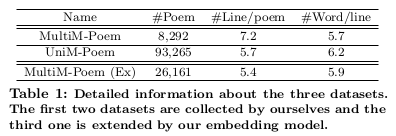1CNN:仅使用对象CNN，通过VGG-16对CNN-RNN模型进行了训练。

3CNNs:使用三个CNN特征，通过VGG-16对CNN-RNN模型进行了训练。

SeqGAN:使用一个判别器（用来分辨生成的诗歌和真人创作的诗歌的判别器）对CNN-RNN模型进行了优化。

BLEU：首先使用双语互译质量评估辅助工具(BLEU) 基于分数的评价来检查生成的诗歌与真实诗歌有多近似，正如图像标题技术和图像生成短文研究通常所做的那样。它还被用于一些其他的诗歌生成研究中， 对于每张图片，仅使用人类创作的诗歌作为真实诗歌。

$\begin{equation} a_i^, = \frac{a_i - min(a)}{max(a) - min(a)} \end{equation}$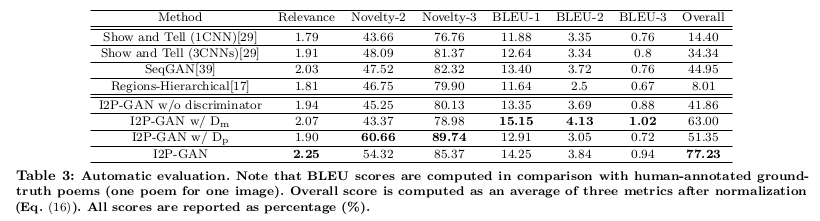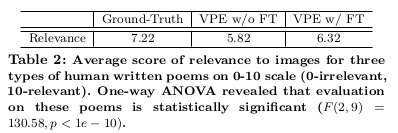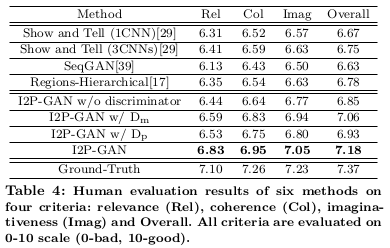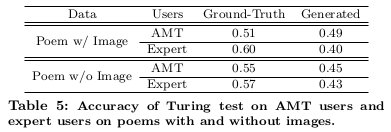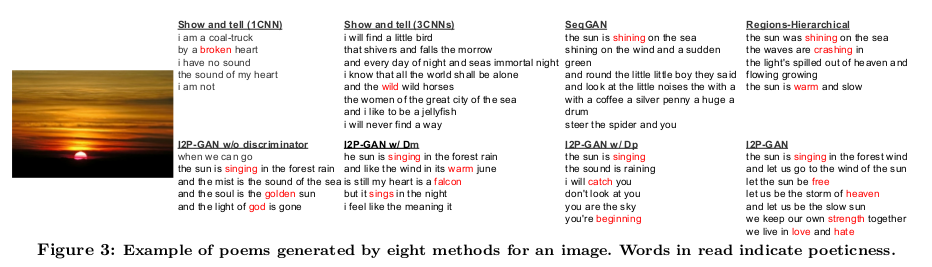# 总结

img2poem是图像生成诗歌（英文自由诗）的首个研究，文章使用多判别器作为策略梯度的奖励，通过整合深度耦合的视觉诗意嵌入模型和基于RNN的对抗训练， 提出了一种模拟问题的新方法。此外，引入了首个图像-诗歌对的数据集（MultiM-Poem）和大型诗歌语料库（UniM-Poem）来促进关于诗歌生成的研究， 特别是根据图像生成诗歌。大量的实验证明，嵌入模型能够近似地学习一个合理的视觉创意嵌入空间。